# Lines Panel

Use the Lines panel to create lines using a wide variety of methods.

Location: Geom page

The Lines panel is organized into many subpanels. Each subpanel is accessed from a toolbar-like row of buttons along the top of the panel. In some cases, a single button can access more then one subpanel; this is indicated by the presence of a small downward arrow (⏷) beside the button. Clicking this arrow reveals a list of possible functions for that button.

On any given subpanel, most of the panel is devoted to options that determine the basis from which the line will be created, whether this is a series of nodes or points, existing lines, surfaces, or mesh features. In all cases the lines are generated by using the green command buttons on the leftmost edge of each subpanel; in most cases these buttons are create and reject, but in some cases they are drag or offset buttons. In all cases, clicking reject undoes the most recent line creation.

Numeric input data, toggles, and switch settings are generally preserved even if you change subpanels or leave the Lines panel altogether; when you return, the settings remain. However, entity selections such as node or line list collectors are cleared when you switch subpanels or return out of the panel.

##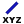xyz Subpanel

Use the XYZ Subpanel to create lines by specifying (x,y,z) coordinates.
Methods for specifying coordinate values include:
• Manually enter the coordinate values into the input boxes.
• Populate individual coordinate values using the x, y and z collectors and selecting a node graphically.

When using each of these collectors, the respective x, y, or z coordinate value is copied from the selected node into the corresponding input box. The values can be edited as desired.

• Populate the coordinate values using the as node collector and selecting a node graphically. This will copy the respective x, y and z coordinate values from the selected node into the corresponding input boxes. The values can be edited as desired.

##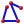Linear Nodes Subpanel

Use the Linear subpanel to create linear lines between nodes.

Two options are required to create a line.
Node list
Select the nodes to use.
A straight line segment is created between each node in the list.
Closed line
Define whether the first and last nodes in the list should be linked to form a closed line loop.

##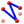Standard Nodes Subpanel

Use the Standard subpanel to create standard lines between nodes, with the possibility of reducing the number of joints and removing some nodes that fall outside of the line's path. The settings for determining joint creation and node removal are preset.

Two options are required to create a line.
Node list
Select the nodes to use.
Closed line
Define whether the first and last nodes in the list should be linked to form a closed line loop.
A standard line is created by performing point reduction using specific parameters.
break angle
Set to 150.0 degrees.
Specify the minimum angle allowed between three points in a line. If the angle between a point and the two adjacent points is less than the angle specified, this point is considered to be a point of discontinuity in the line and a joint is placed at that location.
aspect
Set to 5.0.
Specify the maximum ratio allowed for the distance between a point and the previous point in the line, and the distance between the same point and the next point in the line. If the ratio of the distance between the two adjacent segments exceeds the aspect ratio defined, a joint is placed between the segments.
linear angle
Set to 179.0 degrees.
Specify the angle at which a line is considered a straight line. If the line angle between three consecutive points along the line is greater than the angle specified, the center point is removed from the line.

##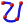Smooth Nodes Subpanel

Use the Smooth subpanel to create smooth lines between nodes.
Four options are required to create a line using this method.
Node list
Select the nodes to uses.
Closed line
Define whether the first and last nodes in the list should be linked to form a closed line loop.
Start slope
Define the line start point constraint. This option does not apply if the closed line option is enabled.
• If set to free, this end point is not constrained.
• If set to normal, this end point is normal to the specified vector.
• If set to vector with tangent to vector selected, this end point is tangent to the specified vector.
• If set to vector with length selected, this end point parametric derivative equals the input vector, assuming a normalized spline parameterization from 0 to 1.
End slope
Define the line end point constraint. This option does not apply if the closed line option is enabled.
• If set to free, this end point is not constrained.
• If set to normal, this end point is normal to the specified vector.
• If set to vector with tangent to vector selected, this end point is tangent to the specified vector.
• If set to vector with length selected, this end point parametric derivative equals the input vector, assuming a normalized spline parameterization from 0 to 1.

A smooth cubic line is created which fits through all of the specified nodes and has the specified start and end slope.

##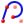Controlled Nodes Subpanel

Use the Controlled Nodes subpanel to specify the values that determine joint creation or node reduction.

This subpanel creates controlled lines between nodes, with the possibility of reducing the number of joints and removing some nodes that fall outside of the line's path. The settings for determining joint creation and node removal are user-specified.

Five options are required to create a line using this method.
Node list
Select the nodes to use.
Closed line
Define whether the first and last nodes in the list should be linked to form a closed line loop.
Break angle
Specify the minimum angle allowed between three points in a line. If the angle between a point and the two adjacent points is less than the angle specified, this point is considered to be a point of discontinuity in the line and a joint is placed at that location.
Aspect
Specify the maximum ratio allowed for the distance between a point and the previous point in the line, and the distance between the same point and the next point in the line. If the ratio of the distance between the two adjacent segments exceeds the aspect ratio defined, a joint is placed between the segments.
Linear angle
Define the angle at which a line is considered a straight line. If the line angle between three consecutive points along the line is greater than the angle specified, the center point is removed from the line.

A controlled line is created by performing point reduction with the specified parameters.

##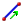Drag Along Vector Subpanel

Use the Drag Along Vector subpanel to create lines by dragging nodes a specified distance along a vector.
Four options are required to create a line using this method.
Nodes
Select the nodes to drag.
Vector
Select a vector defining the direction to drag the node.
Distance
Define the length of the line. This can either be provided directly, or when using the N1/N2/N3 vector definition, by using the distance between N2 and N1.
Drag direction
Define the drag direction.
Drag +
Define using the specified vector direction.
Drag -
Define in the opposite direction.

A line is created at each node by dragging the nodes the specified distance along the given vector.

##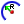Arc Center and Radius Subpanel

Use the Arc Center and Radius subpanel to create arcs by specifying the center and radius. It can create multiple arcs in a single operation.

Five options are required to creat a line using this method.
Node list
Select each arc center. An arc is created around each node in the list.
Plane
Select the plane. Each node in the node list is used as a base node for the plane and the arc is created on that plane.
Specify the radius of each arc. The radius is measured from each node in the node list.
Offset
Specify the offset of each arc. This is an initial offset rotation for the start point of the arc. It is measured from the x-axis of the plane using the right-hand rule.
Angle
Specify the angle of each arc. This is the total angle of the arc. It is measured starting from the offset angle using the right-hand rule.

##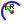Arc Nodes and Vector Subpanel

Use the Arc Nodes and Vector subpanel to create arcs by specifying two nodes and a vector. It can create multiple arcs in a single operation.

Five options are required to create a line using this method.
Node list
Define a location on each arc center. A separate arc is created through each node in the list.
Vector
Define the plane whose normal runs through the center of the arcs and parallel to the plane in which they lie. The base node of the vector defines the arc center.
Offset
Specify the offset of each arc. This is an initial offset rotation for the start point of the arc using the right-hand rule.
Angle
Specify the angle of each arc. This is the total angle of the arc. It is measured starting from the offset angle using the right-hand rule.

##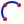Arc Three Nodes Subpanel

Use the Arc Three Nodes subpanel to create arcs by specifying three nodes on the circumference.

Three nodes on the circumference are required to create an arc using this method. The first node defines the start point of the arc and the third node defines the end point of the arc. If more than three nodes are selected, only the first three are used.

Only one arc can be created at a time.

##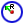Circle Center and Radius Subpanel

Use the Circle Center and Radius subpanel to create circles by specifying the center and radius. Multiple circles can be created in a single operation.

Four options are required to create circles using this method.
Node list
Define a location for each circle center. A circle is created around each node in the list.
Plane
Define a plane. Each node in the node list is used as a base node for the plane and the circle is created on that plane.
Specify the radius of each circle. The radius is measured from each node in the node list.
Offset
Specify the offset of each circle. This is an initial offset rotation for the start point of the circle. It is measured from the x-axis of the plane using the right-hand rule.

##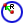Circle Nodes and Vector Subpanel

Use the Circle Nodes and Vector subpanel to create circles by specifying two nodes and a vector.

Three options are required to create a circle using this method.
Node list
Define a location on each circle. A circle is created through each node in the list.
Vector
Define a plane whose normal runs through the center of the circles and parallel to the plane in which they lie. The base node of the vector defines the circle center.
Offset
Specify the offset of each circle. This is an initial offset rotation for the start point of the circle using the right-hand rule.

##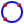Circle Three Nodes Subpanel

Use the Circle Three Nodes subpanel to create circles by specifying three nodes on the circumference.

Three nodes on the circumference are required to create a circle using this method. If more than three nodes are selected, only the first three are used.

Only one circle can be created at a time.

##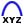Conic Subpanel

Use the Conic subpanel to create conic lines by specifying the start, end and tangent locations.

Four options are required to create a circle using this method.
Start point
Specify coordinates for the start point.
End point
Specify coordinates for the end point.
Tangent point
Specify coordinates for the tangent point, which indicates the point of intersection between straight lines tangent to the created conic curve segment the at start and end points.
Ratio value
Specify the ration value that defines the created conic curve segment “altitude”. Consider the segment that connects the tangents’ intersection point with the midpoint between the start and end points of the curve. If the length of this segment is used as the unit length, then the ratio is defined as the length of the portion of the segment that connects the midpoint with the point where the segment is intersected by created curve. Valid values are in the range 0 to 1. For a ratio of 0.5, the conic curve is a parabolic segment. For values between 0 and 0.5, the conic curve is an ellipse. For values between 0.5 and 1, the conic curve is a hyperbolic segment.
A ratio of -1 can also be used to create a circular arc, provided the length of the tangent segments are equal.

##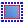Extract Edge Subpanel

Use the Extract Edge subpanel to create lines as copies of surface edges.
Two options are required to create a line using this method.
Line/Surface
Select a line or surface to copy.
If a line is selected, it must be a surface edge.
If a surface is selected, all edges of that surface are considered.
Create in
Choose a method for organizing the resulting lines.
current component
Organize new lines in the current component.
original component
Organize new lines in the same component to which the selected lines or surfaces belong.

##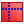Extract Parametric Subpanel

Use the Extract Parametric subpanel to create lines at parametric locations on surfaces.

Eight options are required to create a line using this method.
Surface
Select a surface that the parametric values are in reference to.
Create in
Choose a method for organizing the resulting lines.
current component
Organize new lines in the current component.
surf component
Organize new lines in the same component to which the selected surfaces belong.
Lower u bound
Specify a value that >= 0.0.
Upper u bound
Specify a value that is <= 1.0.
Number of u lines
Specify the number of lines created between the lower and upper u bounds.
If specified as 0, no lines are created along the u direction.
If specified as 1, a line is created at the lower u bound only.
If specified as 2, lines are created at both the upper and lower u bounds.
If specified > 2, lines are created at linear intervals between the lower and upper u bounds.
Lower v bound
Specify a value that is >= 0.0.
Upper v bound
Specify a value that is <= 1.0.
Number of v lines
Specify the number of lines created between the lower and upper v bounds.
If specified as 0, no lines are created along the v direction.
If specified as 1, a line is created at the lower v bound only.
If specified as 2, lines are created at both the upper and lower v bounds.
If specified > 2, lines are created at linear intervals between the lower and upper v bounds.

##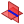Intersect Subpanel

Use the Intersect subpanel to create lines at the intersection of geometric entities (plane/lines, plane/surfaces, plane/elements, plane/plane and surfaces/surfaces).
Geometry type
Select the type of geometry to intersect.
Valid combinations include plane/lines, plane/surfaces, plane/elements, plane/plane and surfaces/surfaces.
Smooth lines
For plane/line intersections, smooth lines determines whether linear segments are created, or whether smooth curves are created.
Line segments
For plane/elems intersections, create a separate line segment for each element. The combine lines option creates single line segments that are combined from the separate segments based on the break angle and smooth lines options.
Break angle
Specify where the intersection line will remain segmented. Segments with an angle relative to each other that exceed this angle will not be combined and will remain separate line segments.
Smooth lines
Create smooth curves within each line segment. The angles between different segments are still not smoothed, but angles less than the break angle are smoothed because segments within the break angle tolerance are combined into a single line segment.

##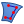Manifold Subpanel

Use the Manifold subpanel to create linear and smooth lines on surfaces using nodes.

Five options are required to create a line using this method.
Surface
Select the surface that the lines will lie on.
Node list
Define the nodes on the surface through which the line will be created. The order of the nodes determines the path of the line.
Trim input surface
Specify if free lines are created or if the input surface is trimmed.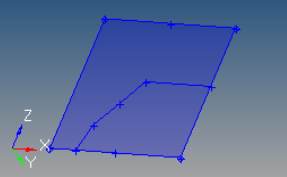Figure 23. . Free lines are coincident with the surface. The surface is not modified.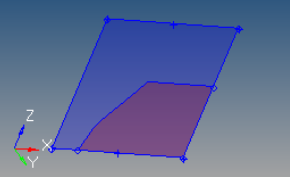Figure 24. . Surface has been split into two different surfaces, one of which is recolored for this example.
Create in
Choose a method for organizing the resulting lines.
current component
Organize new lines in the current component.
surf component
Organize new lines in the same component to which the selected surfaces belong.
Line type
Linear
Create straight line segments between each input node.
Linear closed
Create straight line segments between each input node as well as between the first and last nodes in the list, thereby closing the loop.
Smooth
Create a smooth line between the input nodes.
Smooth closed
Create a smooth line between the input nodes, including closing the loop using the first and last nodes.

##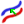Offset Subpanel

Use the Offset subpanel to create lines by offsetting existing curved lines by a uniform or variable distance.

Six options are required to create a line using this method.
Line list
Select the line list to offset.
Create in
Choose a method for organizing the resulting lines.
current component
Organize new lines in the current component.
surf component
Organize new lines in the same component to which the selected lines belong. The result is unpredictable if lines from different components are used as input.
Distance
Define the length to offset the line along its normal.
Uniform
Offset the line list a uniform distance.
Variable
Offset the line list linearly based on a start and end offset value.
Switch start point
The start of the line list is indicated by the end of the chain that has the arrow after selecting the lines, but can be reversed using this option.
Choose how the offset is generated when there is a discontinuity (other than 180 degrees) in the direction of the curvature of the input line list, that is, when input lines do not connect with each other.
interpolate
Interpolate the offset direction on both sides of the discontinuity to allow a smooth transition. In this case, along the interpolation region, the offset direction will be different than the curvature direction. Amplified fluctuations, which would occur in the offset because of small ripples in the input curve, are smoothed out with this option.
Insert a straight line segment as a link between the offset of input lines, if there is a jump in offset direction at points where the input lines meet.
Do not insert a link if there is a jump in offset direction at points where input lines meet. In this case, the offset lines may become disconnected.
Offset direction
Choose the direction to offset.
offset+
Based on the curvature of the selected lines and is shown by an arrow at the start of the line list.
offset-
Defined in the opposite direction.

Closed lines are treated as if they were not closed so that the offset creates open lines.

Successive offsets (4-5 offsets, one after other) or very large offset values of some lines become sensitive to approximation errors or invisible fluctuations in the line.

##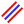Midline Subpanel

Use the Midline subpanel to create lines by interpolating between existing lines.
Two options are required to create a line using this method.
• Select a line list to use for the first side.
• Select a line list to use for the second side.

The result depends on the order of the input lines selection. If the shorter of the two line segments is input as the first list and the longer one as the second, the midline is generated with a length similar to the shorter line. In addition, the midline is created only between the first line segment and a portion of the second line segment.

If the longer line segment is input as the first selection, the length of the midline generated is an average of the two line lengths.

##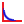Fillet Subpanel

Use the Fillet subpanel to create and delete fillet lines between free lines.

Four options are required to create a fillet using this method.
1st line
Select the first line to fillet.
2nd line
Select the second line to fillet.
Specify the radius of the fillet.
Trim original lines
Specify whether the original input lines are trimmed by the new fillet.

When creating a fillet, the two closest end points are found and the tolerance between them is checked (geometry cleanup tolerance). If the two end points are within the tolerance, the radius must be greater than zero in order to create a fillet. If the two end points are not within the tolerance, and the radius is equal to zero, the closest end points of both lines are extended to their point of intersection, if such a point exists.

If the two selected lines contain at least one curved line, the fillet may not always be placed in the correct quadrant.

If the lines intersect, but not at their end points, the quadrant options are graphically displayed via a set of cross hairs. Pick the appropriate quadrant with the left mouse button.

If the two selected lines intersect in more than one place, the first intersection point is used.

For circular curves, if the arc is smaller than 180 degrees there are no issues. However, if the arc is closer to 360 degrees there may be problems. The algorithm only considers the start and end points of the circular curve to determine where to place the fillet with respect to the selected quadrant. To solve this problem, the curve should be split into segments that are longer than the second line but smaller than 180 degrees in angle of rotation.

One other option on the subpanel is delete line with fillet. This is not directly related to the creation of fillets described above, because the generated fillets are separate line segments. However, single lines that include fillets, such as line and fillet segments that have been combined on the line edit panel, can have their fillet removed by highlighting this option and then selecting the line that contains a fillet.

##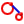Tangent Subpanel

Use the Tangent subpanel to create tangent lines between a line and a node list or line.
Three options are required to create a line using this method.
Node list
Define the tangent origin, or the line that defines the first arc. If multiple nodes are specified for the node list, only the first node is used.
Line
Define the second arc.
Check points
Specify the number of check points to use within each line segment for finding tangents. If the desired tangent is not found, increase the value.

The selected nodes/lines must lie on a plane.

If more than one tangent is found, all the tangent lines found are displayed, and the desired tangent line can be graphically selected.

##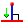Normal to Geometry Subpanel

Use the Normal to Geometry subpanel to create lines perpendicular to existing lines, surfaces and solids from node or point locations.

Four options are required to create a line using this method.
Lines/Surfaces/Solids
Select the lines/surfaces/solids to create the lines perpendicular to.
Nodes/Points
Select the nodes/points that define the starting point locations for the perpendicular lines.
Create normal lines only
Create only perpendicular lines between the node/point and the closest input geometry. No line is created if it would not be perpendicular to the nearest point on the input line.
Clear this checkbox to create the lines between the node/point and the closest input geometry. Lines are not guaranteed to be perpendicular.
Create in
Choose a method for organizing the resulting lines.
current component
Organize new lines in the current component.
input component
Organize new lines in the same component to which the selected lines/surfs/solids belong. The result is unpredictable if geometry from different components are used as input.

A line segment is created for each point/geometry combination.

##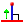Normal from Geometry Subpanel

Use the Normal from Geometry subpanel to create lines perpendicular from node or point locations on lines, surfaces and solids.

Five options are required to create a line using this method.
Lines/Surfaces/Solids
Select the lines/surfaces/solids to create the lines perpendicular from.
Nodes/Points
Select the nodes/points that define the starting point locations for the perpendicular lines. These must be attached/associated to the input geometry.
Create in
Choose a method for organizing the resulting lines.
current component
Organize new lines in the current component.
input component
Organize new lines in the same component to which the selected lines/surfs/solids belong. The result is unpredictable if geometry from different components are used as input.
Length
Specify the length of the lines to create at each location.
Normal line direction
Define the direction of the normal line.
Normal+
Positive normal direction at each node/point location on the geometry.
Normal-
Opposite direction.
A line segment is created for each point/geometry combination.

##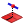Normal 2D on Plane Subpanel

Use the Normal 2D on Plane subpanel to create lines that lie on a plane, are perpendicular to a line, and are defined from node or point locations.
Six options are required to create a line using this method.
Node/Point
Select the node/point that defines the starting point location for the perpendicular line.
Line
Select the line to create the line perpendicular to.
Create in
Choose a method for organizing the resulting lines.
current component
Organize new lines in the current component.
input component
Organize new lines in the same component to which the selected lines/surfs/solids belong. The result is unpredictable if geometry from different components are used as input.
Plane
Define a plane. The new line will lie onn this plane.
Length
Specify a length of the line to create.
Normal line direction
Define the direction of the normal line.
Normal+
Positive normal direction at each node/point location on the geometry.
Normal-
Opposite direction.

The new line has the specified length, is within the specified plane, is perpendicular to the specified line, and starts near the specified node/point.

##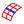Features Subpanel

Use the Features subpanel to create lines from element features.
Five options are required to create a line using this method.
Elems/Comps
Select the elems/components to use as input. If components are selected, the elements in the component are used.
Ignore normals
The direction of the shell face normals are ignored and a feature line is created between any two shell faces whose normals are more than the specified feature angle or less than its inverse (the feature angle minus 180 degrees).
Clear this checkbox to only create a feature line between any two faces whose normals are more than the feature angle, in other words, the inverse angle is not considered.
Feature angle
Specify the maximum angle to allow between the normals of two connected shell elements. When the angle exceeds this value, a feature line is generated.
Break angle
Specify the minimum angle allowed between three points in a line. Line joints will be created whenever the line exceeds this angle.
Smooth lines
Smooth lines.
Clear this checkbox to not smooth lines.